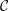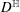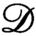Home

### Analytic spaces (32Cxx)

• Article
• ##### On Some Topological Properties of Fourier Transforms of Regular Holonomic${\mathcal{D}}$-Modules
• Canadian Mathematical Bulletin, Volume 63, Issue 2

### Analytic continuation (32Dxx)

• Article
• ##### ON THE OPTIMAL$L^{2}$ EXTENSION THEOREM AND A QUESTION OF OHSAWA
• Nagoya Mathematical Journal, First View

### Holomorphic convexity (32Exx)

• Article
• ##### ON THE OPTIMAL$L^{2}$ EXTENSION THEOREM AND A QUESTION OF OHSAWA
• Nagoya Mathematical Journal, First View

• Article
• #####$L^{p}$ -APPROXIMATION OF HOLOMORPHIC FUNCTIONS ON A CLASS OF CONVEX DOMAINS
• Bulletin of the Australian Mathematical Society, Volume 97, Issue 3

### Deformations of analytic structures (32Gxx)

• Article
• ##### POWER SERIES PROOFS FOR LOCAL STABILITIES OF KÄHLER AND BALANCED STRUCTURES WITH MILD$\partial \overline {\partial }$-LEMMA
• Nagoya Mathematical Journal, First View

### Compact analytic spaces (32Jxx)

• Article
• ##### MINIMAL MODEL THEORY FOR LOG SURFACES IN FUJIKI’S CLASS${\mathcal{C}}$
• Nagoya Mathematical Journal, Volume 244

### Complex spaces with a group of automorphisms (32Mxx)

• Article
• ##### On Motivic Realizations of the Canonical Hermitian Variations of Hodge Structure of Calabi–Yau Type over type$D^{\mathbb{H}}$ Domains
• Canadian Mathematical Bulletin, Volume 62, Issue 1

• Article
• ##### ENDOSCOPY AND COHOMOLOGY IN A TOWER OF CONGRUENCE MANIFOLDS FOR$U(n,1)$
• Forum of Mathematics, Sigma, Volume 7

### Automorphic functions (32Nxx)

• Article
• ##### On Motivic Realizations of the Canonical Hermitian Variations of Hodge Structure of Calabi–Yau Type over type$D^{\mathbb{H}}$ Domains
• Canadian Mathematical Bulletin, Volume 62, Issue 1

• Article
• ##### ENDOSCOPY AND COHOMOLOGY IN A TOWER OF CONGRUENCE MANIFOLDS FOR$U(n,1)$
• Forum of Mathematics, Sigma, Volume 7

### Singularities (32Sxx)

• Article
• ##### Log$\mathscr{D}$-modules and index theorems
• Forum of Mathematics, Sigma, Volume 9

### Pseudoconvex domains (32Txx)

• Article
• #####$L^{p}$ -APPROXIMATION OF HOLOMORPHIC FUNCTIONS ON A CLASS OF CONVEX DOMAINS
• Bulletin of the Australian Mathematical Society, Volume 97, Issue 3

### Pluripotential theory (32Uxx)

• Article
• ##### ON THE OPTIMAL$L^{2}$ EXTENSION THEOREM AND A QUESTION OF OHSAWA
• Nagoya Mathematical Journal, First View

• Article
• ##### WEAK GEODESIC RAYS IN THE SPACE OF KÄHLER POTENTIALS AND THE CLASS${\mathcal{E}}(X,\unicode[STIX]{x1D714})$
• Journal of the Institute of Mathematics of Jussieu, Volume 16, Issue 4

### Differential operators in several variables (32Wxx)

• Article
• ##### ON THE OPTIMAL$L^{2}$ EXTENSION THEOREM AND A QUESTION OF OHSAWA
• Nagoya Mathematical Journal, First View

• Article
• #####$L^{p}$ -APPROXIMATION OF HOLOMORPHIC FUNCTIONS ON A CLASS OF CONVEX DOMAINS
• Bulletin of the Australian Mathematical Society, Volume 97, Issue 3

• Article
• ##### WEAK GEODESIC RAYS IN THE SPACE OF KÄHLER POTENTIALS AND THE CLASS${\mathcal{E}}(X,\unicode[STIX]{x1D714})$
• Journal of the Institute of Mathematics of Jussieu, Volume 16, Issue 4